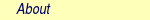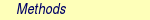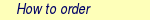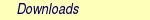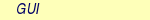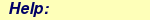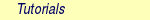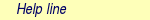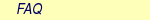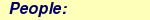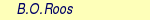MOLCAS manual:Next: 8.4 casvb Up: 8. Programs Previous: 8.2 averd

Subsections

# 8.3 caspt2

Second order multiconfigurational perturbation theory is used in the program CASPT2 [18,19] to compute the (dynamic) correlation energy. The reference state is usually of the CAS type, but the program has been extended to also accept RAS reference states[20,21]. The first step is therefore a RASSCF calculation and the CASPT2 calculation gives a second order estimate of the difference between the RASSCF and the full CI energy. For calculations using a true RAS reference, benchmark calculations were reported by Sauri et al. . For CASSCF references, the CASPT2 method has been tested in a large number of applications [22,23]. Here follows a brief summary of results.

Bond distances are normally obtained with an accuracy of better that 0.01 Å for bonds between first and second row atoms. With the standard Fock matrix formulation, bond energies are normally underestimated with between 2 and 5 kcal/mol for each bond formed. This is due to a systematic error in the method. In every process where the number of paired electrons is changed, an error of this size will occur for each electron pair. For example, the singlet-triplet energy difference in the methylene radical (CH2) is overestimated with about 3 kcal/mol . Heats of reactions for isogyric reactions are predicted with an accuracy of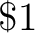kcal/mol. These results have been obtained with saturated basis sets and all valence electrons active. The use of smaller basis sets and other types of active spaces may, of course, affect the error.

These systematic errors have recently been considerably reduced by the introduction of a modified zeroth order Hamiltonian . The method introduces a shift (the IPEA shift) that modifies the energies of active orbitals such that they become closer to ionization energies when excited from and closer to electron affinities when excited out of. The approach has been tested for 49 diatomic molecules, reducing the mean error in D0 from 0.2 to 0.1 eV. For the triply bonded molecules N2, P2, and As2 it was reduced from 0.45 eV to less than 0.15 eV. Similar improvements were obtained for excitation and ionization energies. The IPEA modified H0 (with a shift parameter of 0.25) is default in MOLCAS from version 6.4.

An alternative to IPEA is to use the options, called g1', g2', and g3'(See Ref. ), that stabilizes the energies of the active orbitals. The remaining error is no longer systematic, and is generally reduced. For example, the error in the singlet-triplet separation of CH2 is reduced to 1 kcal/mol . This option is, however, not recommended any longer because it has been replaced by the IPEA Hamiltonian.

The CASPT2 method can be used in any case where a valid reference function can be obtained with the CASSCF method. There is thus no restriction in the number of open shells or the spin coupling of the electrons. Excited states can be treated at the same level as ground states. Actually one of the major successes with the method has been in the calculation of excitation energies. A large number of applications have been performed for conjugated organic molecules. Both Rydberg and valence excited states can be treated and the error in computed excitation energies is normally in the range 0.0-0.2 eV. Similar results have been obtained for ligand field and charge-transfer excitations in transition metal compounds. From MOLCAS-6 it is possible to use the CASPT2 method in conjunction with the Douglas-Kroll-Hess relativistic Hamiltonian, which has made possible calculations on heavy element compounds such a third row transition metal compounds and actinides with accurate results.

The CASPT2 method can also be used in combination with the FFPT program to compute dynamic correlation contributions to properties with good results in most cases. Numerical gradients are available with the slapaf module.

The CASPT2 method is based on second order perturbation theory. To be successful, the perturbation should be small. A correct selection of the active space in the preceding CASSCF calculation is therefore of utmost importance. All near-degeneracy effects leading to configurations with large weights must be included at this stage of the calculation. If this is not done, the first order wave function will contain large coefficients. When this occurs, the CASPT2 program issues a warning. If the energy contribution from such a configuration is large, the results is not to be trusted and a new selection of the active space should be made.

Especially in calculations on excited states, intruder states may occur in the first order wave function. Warnings are then issued by the program that an energy denominator is small or negative. Such intruder states often arise from Rydberg orbitals, which have not been included in the active space. Even if this sometimes leads to large first order CI coefficients, the contribution to the second order energy is usually very small, since the interaction with the intruding Rydberg state is small. It might then be safe to neglect the warning. A safer procedure is to include the Rydberg orbital into the active space. It can sometimes be deleted from the MO space.

Calculations on compounds with heavy atoms (transition metals, actinides, etc) may yield many virtual orbitals with low energies. The interaction energies for excitations to states where these orbitals are occupied are often very small and the low denominators can then be removed by a suitable level shift (see below). But it is always safer to include such orbitals in the active space.

Two keywords have been introduced to deal with this fairly common situation, for excited states, that weakly coupled intruders cause spurious singularities, spikes' in e.g. a potential curve. The two keywords SHIFT and IMAGINARY SHIFT (mutually exclusive) will introduce a shift in the energy denominators, thus avoiding singularities, and will also correct the energy for the use of this shift. The net effect is that the energy is almost unaffected except in the vicinity of the weak singularity, which is removed. The SHIFT keyword adds a real shift, and the use of this procedure is well tested [27,28]. The IMAGINARY SHIFT adds an imaginary quantity, and then uses the real value of the resulting second-order energy . This offers some advantage, in particular for weak intruder states.

In some cases, where one can expect strong interaction between different CASSCF wave functions, it is advisable to use the Multi-State (MS) CASPT2 method . A second order effective Hamiltonian is constructed for a number of CASSCF wave functions obtained in a state-average calculation. This introduces interaction matrix elements at second order between the different CASSCF states. The effective Hamiltonian is diagonalized to obtain the final second order energies. The program also produces a file, JOBMIX, with the new effective zeroth order wave functions, which are linear combinations of the original CASSCF states. This method has been used successfully to separate artificially mixed valence and Rydberg states and for transition metal compounds with low lying excited states of the same symmetry as the ground state.

It is clear from the discussion above that it is not a black box' procedure to perform CASPT2 calculations on excited states. It is often necessary to iterate the procedure with modifications of the active space and the selection of roots in the CASSCF calculation until a stable result is obtained. Normally, the CASSCF calculations are performed as average calculations over the number of electronic states of interest, or a larger number of states. It is imperative that the result is checked before the CASPT2 calculations are performed. The solutions should contain the interesting states. If all of them are not there, the number of roots in the CASSCF calculation has to be increased. Suppose for example, that four states of a given symmetry are required. Two of them are valence excited states and two are Rydberg states. A CASSCF calculation is performed as an average over four roots. Inspection of the solution shows only one valence excited state, the other three are Rydberg states. After several trials it turns out that the second valence excited state occurs as root number seven in the CASSCF calculation. The reason for such a behavior is, of course, the very different dynamic correlation energies of the valence excited states as compared to the Rydberg states. It is important that the AO basis set is chosen to contain a good representation of the Rydberg orbitals, in order to separate them from the valence excited states. For more details on how to perform calculations on excited states we refer to the literature [27,28] and section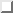of the examples manual.

The first order wave function is obtained in the CASPT2 program as an iterative solution to a large set of linear equations. The size of the equation system is approximately n2*m2/2 where n is the sum of inactive and active orbitals and m is the sum of active and secondary orbitals. Symmetry will reduce the size with approximately a factor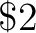, the number of irreps of the point group.

CASPT2 produces a set of molecular orbitals that can be used as start orbitals for other programs or further calculations. A minimal CASSCF and CASPT2 gives orbitals and occupation numbers which can be used to design a proper larger calculation. By default, the orbitals are natural orbitals obtained from the density matrix of the (normalized) wave function through first order. However, the active/active block of that density matrix is not computed exactly. An approximation has been designed in such a way that the trace is correct, and the natural occupation numbers of active orbitals are between zero and two. Due to the approximation, any properties computed using these orbitals are inexact and can be used only qualitatively. An exact first order density matrix can be computed but this is more time-consuming. It is controlled by the keyword DENSity. Use this keyword to compute properties like dipole moments, etc. The most secure accurate way to do that is. however, to use finite field perturbation theory (FFPT).

## 8.3.1 Dependencies

The CASPT2 program needs the JOBIPH file from a RASSCF calculation, and in addition one- and two-electron integrals and some auxiliary files from SEWARD.

## 8.3.2 Files

### 8.3.2.1 Input files

CASPT2 will use the following input files: ONEINT, ORDINT,RUNFILE, JOBIPH (for more information see).

### 8.3.2.2 Output files

 File Contents PT2ORB Molecular orbitals.

## 8.3.3 Input

This section describes the input to the CASPT2 program, starting with its name:

&CASPT2

### 8.3.3.1 Keywords

 Keyword Meaning TITLe This keyword is followed by one title line. MULTistate Enter number of root states, and a list of which CI vector from the CASSCF calculation to use for each state, for example 2 1 2'' would specify the first and second root. Also used for single-state calculations, when the root state is not the ground state, for example 1 2'' would specify the second root. The special value all'' can be used if all the states included in the CASSCF orbital optimization (keyword CIRoot in RASSCF) are desired. IPEAshift This shift corrects the energies of the active orbitals and is specified in atomic units. It will be weighted by a function of the diagonal density matrix element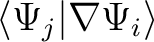. This option is used to modify the standard definition of the zeroth order Hamiltonian (H0), which includes an IPEA shift of 0.25 . The modification of H0 has been introduced (Nov 2005) to reduce the systematic error which leads to a relative overestimation of the correlation energy for open shell system. It also reduces the intruder problems. Default is to use an IPEA shift of 0.25. IMAGinary Add an imaginary shift to the external part of the zero order Hamiltonian. The correlation energy computed is the real part of the resulting complex perturbation energy. Also, a corrected value, obtained by Hylleraas' variational formula, is computed. See Ref. . As with the real shift, this option is used to eliminate intruder problems. SHIFt Add a shift to the external part of the zero order Hamiltonian. See Refs. [29,27,23]. In addition to the conventionally computed second order energy value, another energy obtained by Hylleraas' variational formula is computed. This energy is then very close to the unshifted energy, except close to singularities due to intruders. This option should only be used to eliminate intruder state problems. AFREeze This keyword is used to select atoms for defining the correlation orbital space for the CASPT2 calculation. Assume that you have a large molecule where the activity takes place in a limited region (the active site). It could be a metal atom with its surrounding ligands. You can then use this option to reduce the size of the CASPT2 calculation by freezing and deleting orbitals that have only a small population in the active site. An example: The cobalt imido complex CoIII(nacnac)(NPh) has 43 atoms. The active site was cobalt and the surrounding ligand atoms. Using the AFRE option reduces the time for the CASPT2 calculation from 3 hrs to 3 min with a loss of accuracy in relative energies for 24 electronic states of less than 0.1 eV. The first line after the keyword contains the number of selected atoms then the selection thresholds (the recommended value is 0.1 or less). An additional line gives the names of the atoms as defined in the Seward input. Here is a sample input for the cobalt complex mentioned above. AFREeze   6  0.10  0.00   Co  N1  N2  C5  C6  C7 This input means that inactive orbitals with less than 0.1 of the density on the active sites will be frozen, while no virtual orbitals will be deleted. LOVCaspt2 Freeze-and-Delete'' type of CASPT2, available only in connection with Cholesky or RI. Needs (pseudo)canonical orbitals from RASSCF. An example of input for the keyword LOVC is the following: LovCASPT2   0.3 DoMP2  (or  DoEnv) In this case, both occupied and virtual orbitals (localized by the program) are divided in two groups: those mainly located on the region determined (automatically) by the spatial extent of the active orbitals (active site''), and the remaining ones, which are obviously outside'' this region. The value of the threshold (between 0 and 1) is used to perform this selection (in the example, 30% of the gross Mulliken population of a given orbital on the active site). By default, the CASPT2 calculation is performed only for the correlating orbitals associated with the active site. The keyword DoMP2 is optional and forces the program to perform also an MP2 calculation on the `frozen region''. Alternatively, one can specify the keyword VirAll in order to use all virtual orbitals as correlating space for the occupied orbitals of the active site. A third possibility is to use the keyword DoEnv to compute the energy of the environment as total MP2 energy minus the MP2 energy of the active site. FNOCaspt2 Performs a Frozen Natural Orbital (FNO) CASPT2 calculation, available only in combination with Cholesky or RI integral representation. Needs (pseudo)canonical orbitals from RASSCF. An example of input for the keyword FNOC is the following: FNOCaspt2 0.4 DoMP2 The keyword FNOC has one compulsory argument (real number in ]0,1]) specifying the fraction of virtual orbitals (in each irrep) to be retained in the FNO-CASPT2 calculation. The keyword DoMP2 is optional and used to compute the (estimated) correction for the truncation error. FOCKtype Use an alternative Fock matrix. The default Fock matrix is described in [18,19] and the other original CASPT2 references. The three different modifications named G1, G2 and G3 are described in . Note: from 6.4 it is not recommended to use this keyword but stay with the IPEA modified H0, which is default. FROZen This keyword is used to specify the number of frozen orbitals, i.e. the orbitals that are not correlated in the calculation. The next line contain the number of frozen orbitals per symmetry. The default is to freeze the max of those that were frozen in the RASSCF calculation and the deep core orbitals. The frozen orbitals are always the first ones in each symmetry. DELEted This keyword is used to specify the number of deleted orbitals, i.e. the orbitals that are not used as correlating orbitals in the calculation. The next line contain the number deleted orbitals per symmetry. The default is to delete those that were deleted in the RASSCF calculation. The deleted orbitals are always the last ones in each symmetry. DENSity Computes the full density matrix from the first order wave function, rather than approximated as is the (faster) default option. Used to compute CASPT2 properties, such as dipole moments, etc. RFPErt This keyword makes the program add reaction field effects to the energy calculation. This is done by adding the reaction field effects to the one-electron Hamiltonian as a constant perturbation, i.e. the reaction field effect is not treated self consistently. The perturbation is extracted from RUNOLD, if that file is not present if defaults to RUNFILE. RLXRoot Specifies which root to be relaxed in a geometry optimization of a multi state CASPT2 wave function. Defaults to the highest root or root defined by the same keyword in the RASSCF module. THREsholds On next line, enter two thresholds: for removal of zero-norm components in the first-order perturbed wave function, and for removal of near linear dependencies in the first-order perturbed wave function. Default values are 1.0d-10 and 1.0d-08 respectively. MAXIter On next line, enter the maximum allowed number of iterations in a procedure for solving a system of linear equations using a conjugate gradient method. Default is 20. A gradient norm is reported. This gradient is a residual error from the CASPT2 equation solution and should be small, else the number of iterations must be increased. CONVergence On next line, enter the convergence threshold for the procedure described above. The iterative procedure is repeated until the norm of the residual (RNORM) is less than this convergence threshold. Default is 1.0d-06. NOMIx Normally, a Multi-State CASPT2 calculation produces new jobiph file named JOBMIX. It has the same CASSCF wave functions as the original ones, except that those CI vectors that was used in the Multi-State CASPT2 calculation have been mixed, using the eigenvectors of the effective Hamiltonian matrix as transformation coefficients. Keyword NOMIX prevents creation of this JOBMIX file. NOMUlt This keyword removes the multi-state part of the calculation and only runs a series of independent CASPT2 calculations for the roots specified by the MULTistate keyword. Useful when many roots are required, but multi-state is not needed, or desired. Note that a JOBMIX file is produced anyway, but the vectors will not be mixed, and the energies will be single-state CASPT2 energies. ONLY This keyword requires the MULTistate keyword, and is followed by an integer specifying one of the roots. In a Multistate calculation, it requests to compute the energy of only the specified root. However, the effective Hamiltonian coupling terms bewteen this root and all the others included in the Multistate treatement will be computed and printed out. This output will be used in a subsequent calculation, in conjuction with the EFFE keyword. EFFE This keyword requires the MULTistate keyword. It is followed by a matrix of real numbers, specifying the effective Hamiltonian couplings, as provided in a previous calculation using the ONLY keyword. In a Multistate calculation over, e.g., 3 states, 3 separate calculations with the ONLY keyword will be performed, possibly on separate computing nodes, so as to speed up the overall process. The three couplings vectors will be given to the EFFE keyword in matrix form, i.e. the first column is made by the couplings of the first computed root, etc. The program will then quickly compute the Multistate energies. NOORbitals In calculations with very many orbitals, use this keyword to skip the printing of the MO orbitals. PROPerties Normally, a CASPT2 calculation does not produce any density matrix, natural orbitals or properties in order to save time and memory (especially for large calculations). Keyword PROP activates these calculations, at the expense of (some) extra time and memory (especially if used together with the DENS keyword). NOTRansform This keyword specifies that the wave function should not be transformed to use quasi-canonical orbitals, even if CASPT2 does not know if this was done or not and by default would do such a transformation. Effectively, the Fock matrix is replaced by a diagonal approximation in the input orbital system. TRANsform This keyword specifies that the wave function should be transformed to use pseudo-canonical orbitals, even if this was specified as option to the CASSCF calculation and should be unnecessary. (Default is: to transform when necessary, and not else.) OFEMbedding Adds an Orbital-Free Embedding potential to the hamiltonian. Available only in combination with Cholesky or RI integral representation. No arguments required. The runfile of the environment subsystem (AUXRFIL) must be available. GHOStdelete Excludes from PT2 treatment orbitals localized on ghost atoms. A threshold for this selection must be specified. OUTPut Use this keyword, followed by any of the words BRIEF, DEFAULT, or LONG, to control the extent of orbital listing. BRIEF gives a very short orbital listing, DEFAULT a normal output, and LONG a detailed listing. PRWF This keyword is used to specify the threshold for printing the CI coefficients, default is 0.05. PRSD This keyword is used to request that not only CSFs are printed with the CI coefficients, but also the determinant expansion.

The given default values for the keywords Convergence and Thresholds normally give a second order energy which is correct in eight decimal places.

### 8.3.3.2 Input example

&CASPT2
Title
The  water  molecule
Density  matrix
The CASPT2 energy and density matrix is computed for the water molecule with the O(1s) orbital frozen. The standard IPEA-H0 is used.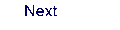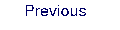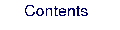Next: 8.4 casvb Up: 8. Programs Previous: 8.2 averd